Pandas Dataframe Examples: Manipulating Date and Time

Last updated:

For information on the advanced Indexes available on pandas, see Pandas Time Series Examples: DatetimeIndex, PeriodIndex and TimedeltaIndex

Convert column to date/datetime

Use pd.to_datetime(string_column):

import pandas as pd

df = pd.DataFrame({
'name': ['alice','bob','charlie'],
'date_of_birth': ['10/25/2005','10/29/2002','01/01/2001']
})

df['date_of_birth'] = pd.to_datetime(df['date_of_birth'])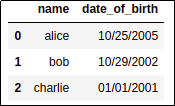BEFORE: column is of type 'object'AFTER: column 'date_of_birth' is
now of type 'datetime' and you can
perform date arithmetic on it

For custom formats, use format parameter:

See all formats here: python strftime formats

import pandas as pd

df = pd.DataFrame({
'name': ['alice','bob','charlie'],
'date_of_birth': ['27/05/2001','16/02/1999','25/09/1998']
})

df['date_of_birth'] = pd.to_datetime(df['date_of_birth'],format='%d/%m/%Y')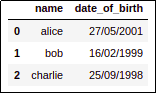BEFORE: In some countries, dates are often
displayed in a day/month/year
format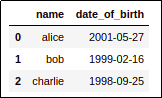AFTER: Just pass the format parameter so that
pandas knows what format your
dates are in

Filter rows where date smaller than X

Only works for columns of type datetime (see above)

Use pandas.Timestamp(<date_obj>) to create a Timestamp object:

import pandas as pd

from datetime import date

df = pd.DataFrame({
'name': ['alice','bob','charlie'],
'date_of_birth': ['10/25/2005','10/29/2002','01/01/2001']
})

# convert to type datetime
df['date_of_birth'] = pd.to_datetime(df['date_of_birth'])

df[df['date_of_birth'] < pd.Timestamp(date(2002,1,1))]

dfBEFORE: original dataframe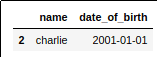AFTER: only charlie was born prior to 1/1/2002

Filter rows where date in range

import pandas as pd
from datetime import date

date_from = pd.Timestamp(date(2003,1,1))
date_to = pd.Timestamp(date(2006,1,1))

# df is defined in the previous example

df = df[
(df['date_of_birth'] > date_from ) &
(df['date_of_birth'] < date_to)
]

dfBEFORE: original dataframe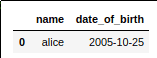AFTER: only alice's date of birth is
between 2003/01/01 and 2006/01/01

Group by year

Naturally, this can be used for grouping by month, day of week, etc

Create a column called 'year_of_birth' using function strftime and group by that column:

# df is defined in the previous example

# step 1: create a 'year' column
df['year_of_birth'] = df['date_of_birth'].map(lambda x: x.strftime('%Y'))

# step 2: group by the created columns
grouped_df = df.groupby('year_of_birth').size()

grouped_df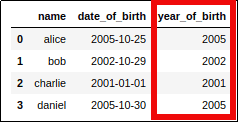STEP 1: Add a new column to
the original dataframe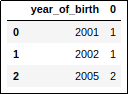STEP 2: number of records per year